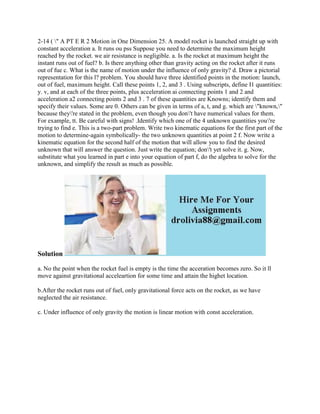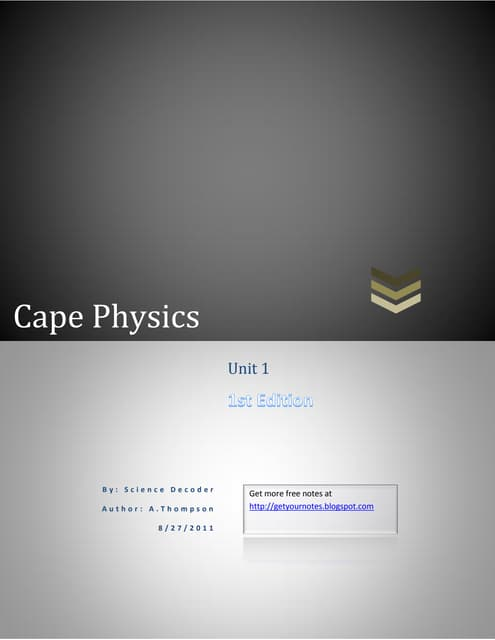AnúncioPróximos SlideSharesCape physics unit 1st edition
Carregando em ... 3
1 de 2
Anúncio

### 2-14 ( -- A PT E R 2 Motion in One Dimension 25- A model rocket is lau.docx

1. 2-14 ( " A PT E R 2 Motion in One Dimension 25. A model rocket is launched straight up with constant acceleration a. It runs ou pss Suppose you need to determine the maximum height reached by the rocket. we air resistance is negligible. a. Is the rocket at maximum height the instant runs out of fuel? b. Is there anything other than gravity acting on the rocket after it runs out of fue c. What is the name of motion under the influence of only gravity? d. Draw a pictorial representation for this l? problem. You should have three identified points in the motion: launch, out of fuel, maximum height. Call these points 1, 2, and 3 . Using subscripts, define I1 quantities: y. v, and at each of the three points, plus acceleration ai connecting points 1 and 2 and acceleration a2 connecting points 2 and 3 . 7 of these quantities are Knowns; identify them and specify their values. Some are 0. Others can be given in terms of a, t, and g. which are "known," because they're stated in the problem, even though you don't have numerical values for them. For example, tt. Be careful with signs! .Identify which one of the 4 unknown quantities you're trying to find e. This is a two-part problem. Write two kinematic equations for the first part of the motion to determine-again symbolically- the two unknown quantities at point 2 f. Now write a kinematic equation for the second half of the motion that will allow you to find the desired unknown that will answer the question. Just write the equation; don't yet solve it. g. Now, substitute what you learned in part e into your equation of part f, do the algebra to solve for the unknown, and simplify the result as much as possible. Solution a. No the point when the rocket fuel is empty is the time the acceration becomes zero. So it ll move against gravitational acceleartion for some time and attain the highet location. b.After the rocket runs out of fuel, only gravitational force acts on the rocket, as we have neglected the air resistance. c. Under influence of only gravity the motion is linear motion with const acceleration.
Anúncio